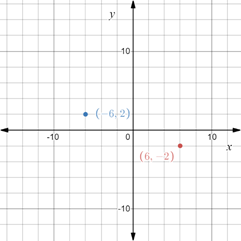# To graph : The points ( 6 , − 2 ) and ( − 6 , 2 ) in a coordinate plane.### Precalculus: Mathematics for Calcu...

6th Edition
Stewart + 5 others
Publisher: Cengage Learning
ISBN: 9780840068071### Precalculus: Mathematics for Calcu...

6th Edition
Stewart + 5 others
Publisher: Cengage Learning
ISBN: 9780840068071

#### Solutions

Chapter 1.8, Problem 17E

(a)

To determine

## To graph: The points (6,−2) and (−6,2) in a coordinate plane.

Expert Solution

### Explanation of Solution

Given information:

The points, (6,2) and (6,2) .

Graph:

The plot the points (6,2) and (6,2) in the coordinate plane.

Construct a Cartesian plane with vertical axis as y-axis and horizontal axis as x-axis.

Marks the numbers on both the axes.

Plot the points (6,2) and (6,2) .Interpretation:

The Cartesian plane is divided into four quadrant. Numbers on right of zero are positive, on left of zero are negative. Numbers above zero on vertical line are positive and numbers below 0 are negative. Each pair of point in the coordinate plane is represented as an ordered pair (x,y) where first element is the x-coordinate and second element is the y-coordinate. The point (x,y) denote it is x units on x-axis and y units on y −axis and marked accordingly.

(b)

To determine

### To calculate: The distance between the pair of points (6,−2) and (−6,2) .

Expert Solution

The distance between the pair of points (6,2) and (6,2) is 410 units.

### Explanation of Solution

Given information:

The points (6,2) and (6,2) .

Formula used:

Distance d(A,B) between two points A=(x1,y1) and B=(x2,y2) in the Cartesian plane is denoted by d(A,B)=(x2x1)2+(y2y1)2 .

Calculation:

Consider the points (6,2) and (6,2) .

Recall that the distance d(A,B) between two points A=(x1,y1) and B=(x2,y2) in the Cartesian plane is denoted by d(A,B)=(x2x1)2+(y2y1)2 .

Evaluate the distance between (6,2) and (6,2) .

d(A,B)=(66)2+(2(2))2=144+16=160=410

Thus, the distance between the pair of points is 410 units.

(c)

To determine

### To calculate: The midpoint of the segment that joins the pair of points (6,−2) and (−6,2) .

Expert Solution

The midpoint of the segment that joins the pair of points (6,2) and (6,2) is (0,0) .

### Explanation of Solution

Given information:

The points are (6,2) and (6,2) .

Formula used:

Midpoint of the segment that joins two points A=(x1,y1) and B=(x2,y2) in the Cartesian plane is denoted by (x1+x22,y1+y22) .

Calculation:

Consider the points (6,2) and (6,2) .

Recall that the midpoint of the segment that joins two points A=(x1,y1) and B=(x2,y2) in the Cartesian plane is denoted by (x1+x22,y1+y22) .

Evaluate the midpoint of the segment that joins (6,2) and (6,2) .

(x1+x22,y1+y22)=(662,2+22)=(02,02)=(0,0)

Thus, the midpoint of the segment that joins the pair of points is (0,0) .

### Have a homework question?

Subscribe to bartleby learn! Ask subject matter experts 30 homework questions each month. Plus, you’ll have access to millions of step-by-step textbook answers!